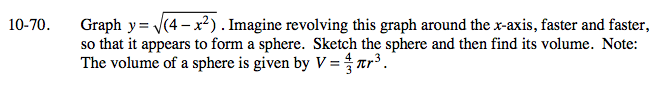### Home > A2C > Chapter Ch10 > Lesson 10.2.1 > Problem10-70

10-70.Square both sides of the first equation and convert it into graphing form. What is the radius of the sphere?

$\text{Volume }=\frac{32\pi}{3}$